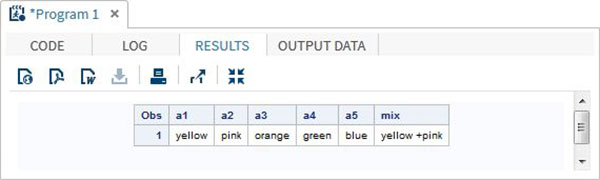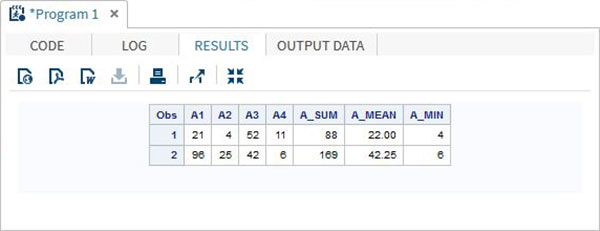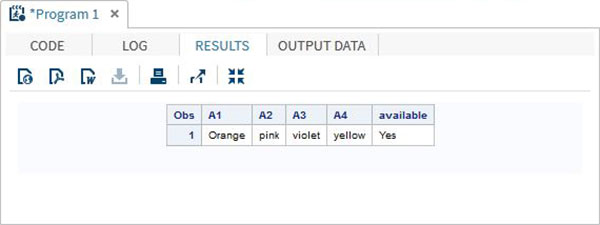# SAS - Arrays

Arrays in SAS are used to store and retrieve a series of values using an index value. The index represents the location in a reserved memory area.

## Syntax

In SAS an array is declared by using the following syntax −

```ARRAY ARRAY-NAME(SUBSCRIPT) (\$) VARIABLE-LIST ARRAY-VALUES
```

In the above syntax −

• ARRAY is the SAS keyword to declare an array.

• ARRAY-NAME is the name of the array which follows the same rule as variable names.

• SUBSCRIPT is the number of values the array is going to store.

• (\$) is an optional parameter to be used only if the array is going to store character values.

• VARIABLE-LIST is the optional list of variables which are the place holders for array values.

• ARRAY-VALUES are the actual values that are stored in the array. They can be declared here or can be read from a file or dataline.

### Examples of Array Declaration

Arrays can be declared in many ways using the above syntax. Below are the examples.

```# Declare an array of length 5 named AGE with values.
ARRAY AGE (12 18 5 62 44);

# Declare an array of length 5 named COUNTRIES with values starting at index 0.
ARRAY COUNTRIES(0:8) A B C D E F G H I;

# Declare an array of length 5 named QUESTS which contain character values.
ARRAY QUESTS(1:5) \$ Q1-Q5;

# Declare an array of required length as per the number of values supplied.
```

## Accessing Array Values

The values stored in an array can be accessed by using the print procedure as shown below. After it is declared using one of the above methods, the data is supplied using DATALINES statement.

```DATA array_example;
INPUT a1 \$ a2 \$ a3 \$ a4 \$ a5 \$;
ARRAY colours(5) \$ a1-a5;
mix = a1||'+'||a2;
DATALINES;
yello pink orange green blue
;
RUN;
PROC PRINT DATA = array_example;
RUN;
```

When we execute above code, it produces following result −### Using the OF operator

The OF operator is used when analysing the data forma an Array to perform calculations on the entire row of an array. In the below example we apply the Sum and Mean of values in each row.

```DATA array_example_OF;
INPUT A1 A2 A3 A4;
ARRAY A(4) A1-A4;
A_SUM = SUM(OF A(*));
A_MEAN = MEAN(OF A(*));
A_MIN = MIN(OF A(*));
DATALINES;
21 4 52 11
96 25 42 6
;
RUN;
PROC PRINT DATA = array_example_OF;
RUN;
```

When we execute above code, it produces following result −### Using the IN operator

The value in an array can also be accessed using the IN operator which checks for the presence of a value in the row of the array. In the below example we check for the availability of the colour "Yellow" in the data. This value is case sensitive.

```DATA array_in_example;
INPUT A1 \$ A2 \$ A3 \$ A4 \$;
ARRAY COLOURS(4) A1-A4;
IF 'yellow' IN COLOURS THEN available = 'Yes';ELSE available = 'No';
DATALINES;
Orange pink violet yellow
;
RUN;
PROC PRINT DATA = array_in_example;
RUN;
```

When we execute above code, it produces following result −